# Divide In ExcelArticle byExcelMojo Team## What Is Divide In Excel?

Divide in Excel is a formula that performs the arithmetic operation of division. It divides two given numbers to determine the quotient. Users can use the division formula in Excel to perform calculations such as dividing columns, a column by a numeric value, and a value by percentage.

For example, the below table contains values’ sets and the descriptions to execute division formulas using the values in the corresponding rows.

Let us assume the requirement is to perform the divisions along with the other arithmetic operations in column D. Then, ‘/’ (Forward Slash), the symbol for divide in Excel, can help perform the division operation in the target cells.

###### Key Takeaways
• Divide in Excel performs the division arithmetic operation. It divides the specified values, such as numbers, cell references to numbers, or formulas returning numbers.
• Users can use the division formula to divide columns or rows, a column or row by a number, and a value by percentage.
• We can use the Forward Slash (‘/’) or the QUOTIENT() to perform the division operation. While using the Forward Slash to divide values will return the quotient as is, the QUOTIENT() will return the integer part of the resulting quotient.
• The Excel functions IF and IFERROR help overcome the #DIV/0! error.

### How To Divide In Excel?

The steps to divide in Excel are as follows:

1. First, confirm the values to divide are valid and accurate.
2. Next, select the target cell and type the division formula.
3. Finally, press Enter to view the division result.

Excel does not provide an inbuilt division function. However, we can divide in Excel using the following methods.

We can perform the division operation using the ‘/’ (Forward Slash), the symbol for divide in Excel.

For example, to enter a straightforward division formula for dividing two numbers, say, N1 and N2, we must enter the ‘=’, then N1, ‘/’, N2, without any spaces.

Ensure we start the expression with the ‘=’. Otherwise, Excel might consider the entered expression to be a date value.

When we divide in Excel, the number in the numerator is the dividend (The value we require to divide). And the number in the denominator is the divisor, which divides the dividend.

Meanwhile, when we supply the numerator and denominator values directly in the division formula, providing the excel cell references to the numbers also works.

Let us see the above scenarios with an example.

The following table contains values to divide according to the specified descriptions.

Suppose we must create the division formulas and execute them in column D. Then, we can get the required results using the divide sign in the target cells.

Steps to divide in excel are as follows –

1. First, select the target cell D2, enter the below formula, and press Enter.

=110/4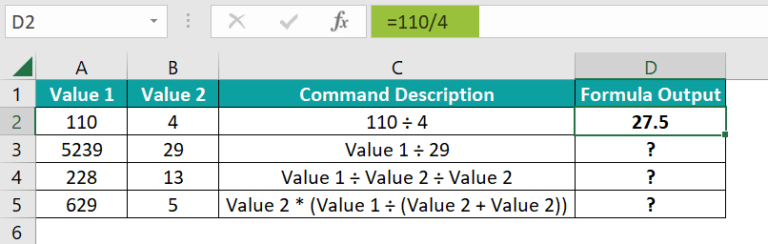2. Next, select the target cell D3, enter the below formula, and press Enter.

=A3/29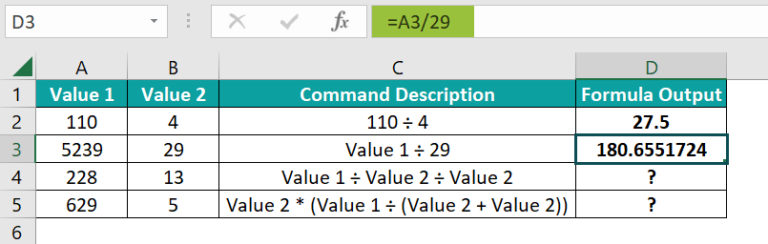3. Then, select the target cell D4, enter the below formula to divide multiple cells, and press Enter.

=A4/B4/B4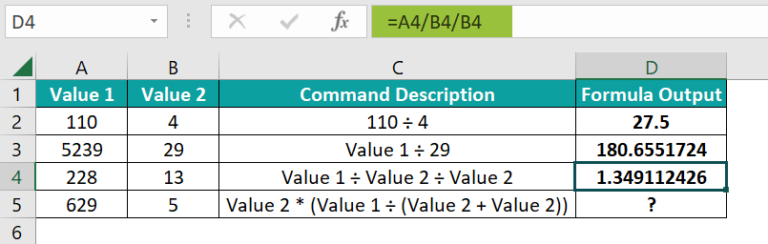4. Select the target cell D5, enter the below formula, and press Enter.

=B5*(A5/(B5+B5))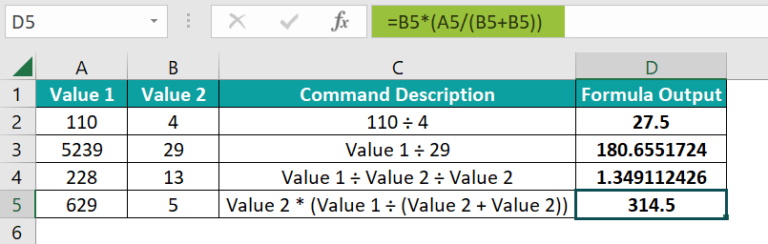Thus, the division formulas in column D show how to use the ‘/’ to perform the division operation (represented by the symbol, ‘÷’, in the command descriptions). While some formulas use the input numbers directly, others use cell references to the numbers.

However, ensure to use the divide sign at the right position in the formula because Excel follows the PEDMAS order of arithmetic operations. The execution order is parentheses, exponentiations, division or multiplication (whichever occurs first), and addition or subtraction (whichever occurs first).

### Divide Function In Excel (QUOTIENT)

The QUOTIENT() helps us perform divide in Excel. But the function is useful when we require to divide two numbers and need only the integer part of the resulting quotient.

The QUOTIENT() syntax is:

where

• numerator: It is the dividend.
• denominator: It is the divisor.

Remember, the two arguments in the above QUOTIENT() syntax are mandatory.

Further, adhering to the below points will ensure to avoid the condition of the divide in excel not working.

• We can supply numbers, cell references to numbers, or formulas returning numbers as the QUOTIENT() arguments.
• For non-numeric argument values, either one of them or both, the QUOTIENT() throws the #VALUE! error.
• Remember, for 0 supplied as the denominator, the QUOTIENT() returns the #DIV/0! error.

The following example explains how to use QUOTIENT().

The first table contains the values to divide, and the second table shows the division formulas using the divide sign, ‘/’.

Suppose the requirement is to use QUOTIENT() to divide the numbers given in the first table and display the results in cell range D6:D7. Then, the steps are as follows:

• Step 1: To begin with, select the target cell D6, enter the QUOTIENT(), and press Enter.

=QUOTIENT(A2,B2)

Next, enter the formula in cell D7 using the fill handle in excel.

The above example shows the division formulas in cells D2 and D6 return the same result, 8. The reason is that the division of 80000 by 10000 gives a 0 remainder.

On the other hand, the division formulas in cells D3 and D7 return different results, 11.09638554 and 11, for the same input values. It is because dividing 921 by 83 gives a remainder of 8. So, using the divide sign to divide the given numbers gives the quotient as is, 11.09638554. But the QUOTIENT() divides the given numbers and returns the integer portion of the quotient, 11.

### How To Divide Columns In Excel?

We can divide columns in Excel in the following ways:

• First, enter the division formula that divides the two specified column cells’ values in the first cell of the target column. And then, copy the formula into the remaining target cells.
• Next, execute the expression to divide in Excel as an array excel formula.

Let us see the two methods with an example.

The table below contains five employees’ monthly salaries and the number of working days.

Suppose the requirement is to determine the per-day salary of each employee and display the result in column D. Then, applying the division formula in the target cells will help us obtain the required data.

Assume the target cell range D2:D6 has the data format set as Currency in the HomeNumber Format option.

• Step 1: First, select the target cell D2, enter the following division formula, and press Enter.

=B2/C2

• Step 2: Next, using the fill handle, enter the division formula in cell range D3:D6.

Each division formula in cell range D2:D6 divides the monthly salary by the total days worked in a month to return the per-day wages of each employee.

Alternatively, we can apply the above formula as an array formula.

• Step 1: To begin with, select the cell range D2:D6 and enter the division formulas as explained below.

=B2:B6/C2:C6

Next, press the Ctrl + Shift + Enter keys to execute the above expression as an array formula.

{=B2:B6/C2:C6}

In the above array formula, the numerator and denominator are arrays, and the formula divides them to return the per-day wages of each employee in cell range D2:D6.

### How To Divide A Column By A Number?

The below methods can help us divide a column by a number:

• First, supply the number directly as the denominator to divide the column cells with the number.
• Next, supply the cell reference to the number as the denominator to divide the column cells with the number.
• Then, use the Paste Special feature to divide in Excel.

Here is an example that explains the above methods.

The first table contains a list of items, and their weekly units delivered data.

Suppose we must determine the units delivered on each day of the week for the five items.

Then, the first way to get the required data is to divide each weekly unit delivered data by 7 (total number of days in a week). Assume the target cells are cell range D3:D7.

• Step 1: To start with, select the target cell D3, enter the following formula, and press Enter.

=B3/7

Next, use the fill handle to enter the formula in cell range D4:D7.

The second way is to provide the denominator in the division formula as the absolute excel reference to the cell containing the required denominator value, 7.

Thus, it is an example of the divide in Excel with absolute reference. And in this case, the denominator is cell reference, \$G\$1. Assume the target cell range is F3:F7

• Step 1: To begin with, select the target cells F3, enter the below formula, and then, press Enter.

=B3/\$G\$1

Next, use the fill handle to implement the above formula for the divide in Excel with absolute reference in cell range F4:F7.

The absolute reference to the denominator value of 7 ensures it remains constant in all the target cells.

Next, let us modify the source table to see how to use the Paste Special feature to divide in Excel.

Now, we shall introduce a new target column to determine the units delivered per day for each item and copy column B values into it. Also, let us enter the denominator value of 7 in cell F1.

Next, we shall follow the below process to achieve the required data in the target cells.

• Step 1: Select cell F1, press Ctrl + C to copy the cell value, and choose the cell range C2:C6.
• Step 2: Press the keys Ctrl + Alt + V to open the Paste Special window. And then, press the key I to select the Divide option.

And once we click OK, the Paste Special window will close, and then, we will see column C updated with the required data.

The above steps will divide each column B cell value in the cell range B2:B6 with the cell F1 value, 7, to give the required per day units delivered count for each item. However, as we use the Paste Special feature, the target cells will show only the values and not the formulas.

### How To Divide By Percentage In Excel?

The following steps show how to divide by percentage in Excel.

1. First, ensure we supply the denominator in a division formula as a percentage or the decimal equivalent.
2. Next, select the required cell and enter the formula for the divide in Excel.
3. Finally, press Enter to view the division result.

For example, the below table contains two sets of numerators and denominators.

The numerator in both sets are same, 725. And the denominator in the first and second sets are a percentage, 85%, and its decimal equivalent, 0.85, respectively.

Suppose the requirement is to use the values in each set to divide in Excel and round up the result in column C.

Then, the steps are:

• Step 1: First, select the target cell C2, enter the below formula, and then press Enter.

=ROUNDUP(A2/B2,0)

Alternatively, entering the denominator directly as the percentage will give the same result.

=ROUNDUP(A2/85%,0)

• Step 2: Next, select the target cell C3, enter the below formula, and then, press Enter.

=ROUNDUP(A2/B3,0)

In the above divide in Excel and round up formulas, first, the number argument expression in the ROUNDUP() divides the numbers specified in the corresponding rows. And then, the ROUNDUP() rounds up the division result to 0 digits, away from 0.

Since the numerator and denominator values in both sets are the same, the corresponding target cells show the same rounded-up quotient value of 853.

### How To Handle #DIV/0! Error In Excel Divide

Getting the #DIV/0! error indicates the scenario of the divide in Excel not working. And it occurs when the denominator value is 0.

However, providing the division formula inside the IF or IFERROR excel functions can help us overcome the #DIV/0! error.

For example, the below table shows the total stock price and units purchased data for two stocks.

Suppose the requirement is to calculate the per unit price of each stock and display the output in cell range D2:D3. But, as the column C data (denominator values) is missing, applying the formulas to perform divide in Excel cells, D2:D3, will return the #DIV/0! error.

Thus, here is how we can avoid displaying the above error value.

• Step 1: Select the target cell D2, enter the below IF(), and press Enter.

=IF(C2=0,”Insufficient Data”,B2/C2)

Alternatively, entering the following IF() will return the same output.

=IF(C2<>0,B2/C2,”Insufficient Data”)

The two IF functions check whether the supplied denominator value is 0 or not 0, respectively. And if the first IF() condition holds or the second IF() condition is false, the result in the target cell is the message, “Insufficient Data”, to replace the #DIV/0! error.

• Step 2: Select the target cell D3, enter IFERROR(), and press Enter.

=IFERROR(B3/C3,”Insufficient Data”)

In the above formula, the value argument expression in the IFERROR() divides the specified numbers. And as the division operation results in the #DIV/0! error, the IFERROR() replaces the error value with the text “Insufficient Data”.

### Important Things To Note

• When using the Forward Slash (‘/’) to divide in Excel, ensure we start entering the formula with the ‘=’ sign. Otherwise, Excel will consider the cell content as a date value.
• When using the Forward Slash (‘/’) with other arithmetic operators in a formula, ensure you place it in the correct position. The reason is that Excel follows the PEDMAS rule while executing arithmetic operations.
• Dividing non-numeric values will return the #VALUE! error.
• Dividing a number with 0 will result in the #DIV/0! error.

1. Is there a Divide function in Excel?

There is no Divide function in Excel. However, we can divide the specified values using the Forward Slash (‘/‘).

Further, we can apply QUOTIENT() to perform the division operation. However, the function will return only the integer part of the resulting quotient.

2. How to apply the divide formula in Excel VBA?

We can apply the divide formula in Excel VBA using the Forward Slash (‘/’).
Let us see the steps with an example.

The following table contains the yearly estimated and actual costs of a project.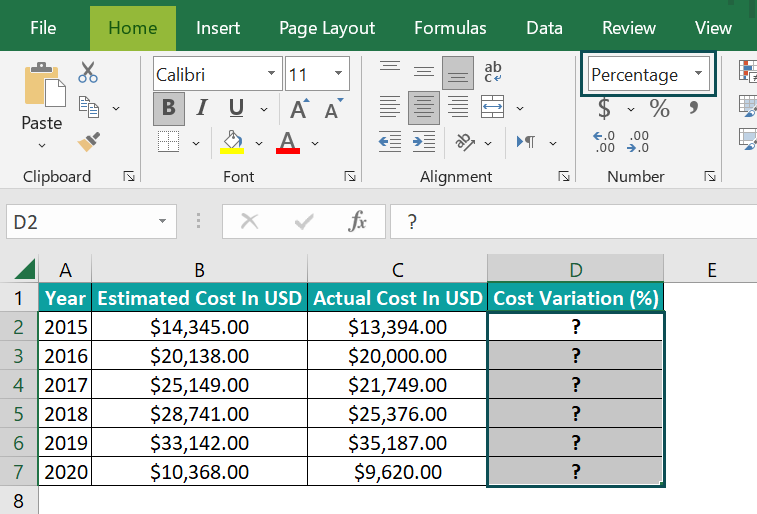Suppose we must find the yearly cost variations in percentage and display the results in column D. Then, the steps to perform the necessary calculations involving the division operations in Excel VBA and get the output in the target cells are as follows:

Assume the target cells’ data format set using HomeNumber Format is Percentage.

• Step 1: Keep the active worksheet containing the above table open and press Alt + F11 to open the VBA Editor.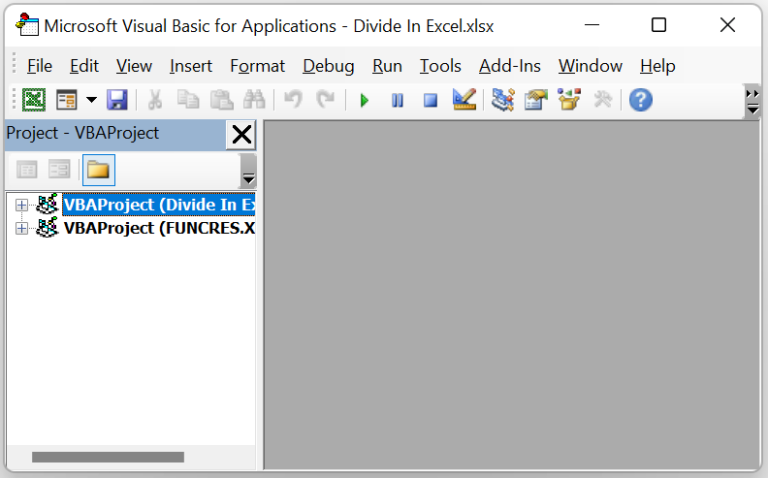• Step 2: Pick the required VBAProject and choose the tab Insert 🡪 Module option to open a new module window.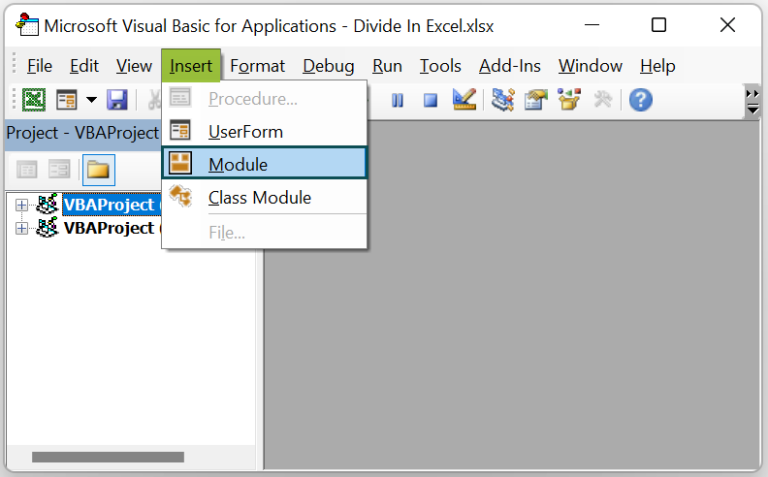• Step 3: Enter the VBA code in the module window to execute the required formulas in the target cells.

Sub Divide_fn()
Dim ws As Worksheet
Set ws = Worksheets(“FAQ_2”)
ws.Range(“D2”) = (ws.Range(“B2”) – ws.Range(“C2”)) / ws. Range(“B2”)
ws.Range(“D3”) = (ws.Range(“B3”) – ws.Range(“C3”)) / ws. Range(“B3”)
ws.Range(“D4”) = (ws.Range(“B4”) – ws.Range(“C4”)) / ws. Range(“B4”)
ws.Range(“D5”) = (ws.Range(“B5”) – ws.Range(“C5”)) / ws. Range(“B5”)
ws.Range(“D6”) = (ws.Range(“B6”) – ws.Range(“C6”)) / ws. Range(“B6”)
ws.Range(“D7”) = (ws.Range(“B7”) – ws.Range(“C7”)) / ws. Range(“B7”)
End Sub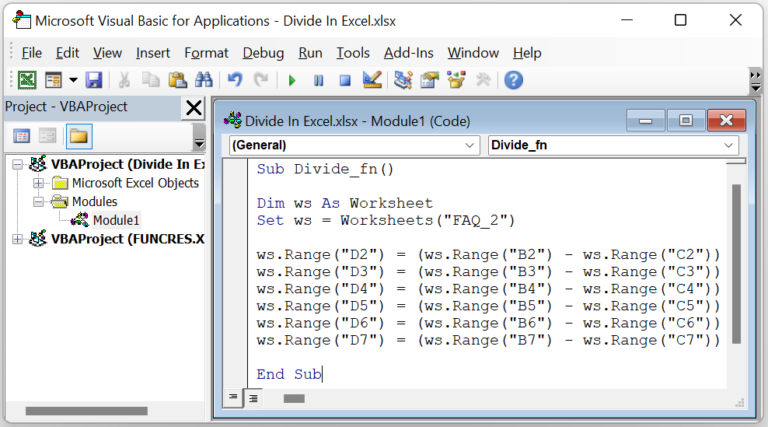• Step 4: Click the Run Sub/UserForm icon to execute the VBA code.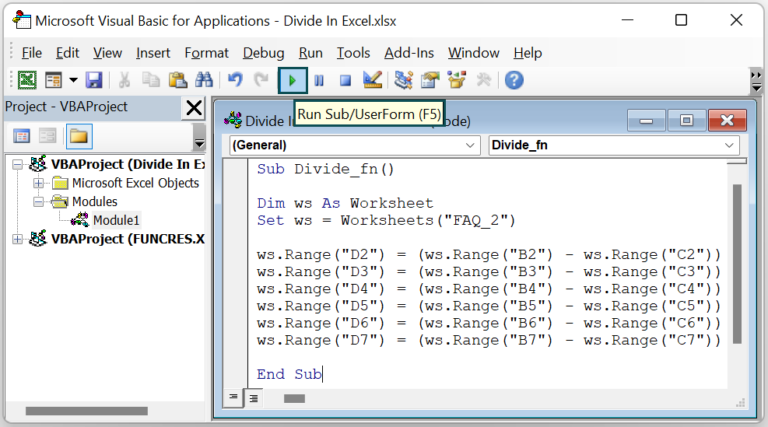We can now open the table to view the results.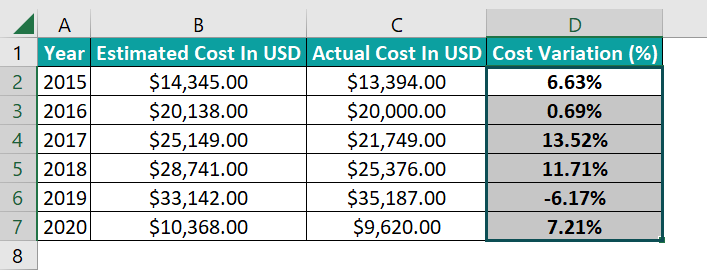The above code determines the cost variation using the below formula:
=(Estimated Cost – Actual Cost)/Estimated Cost

And the code uses ‘/‘ to perform the division operation in each formula assigned to the target cells.

3. How to divide rows by a number?

We can divide rows by a number using the Forward Slash (‘/’).

Let us see the steps with an example.
The below table contains the 2018-20 sales figures of five companies.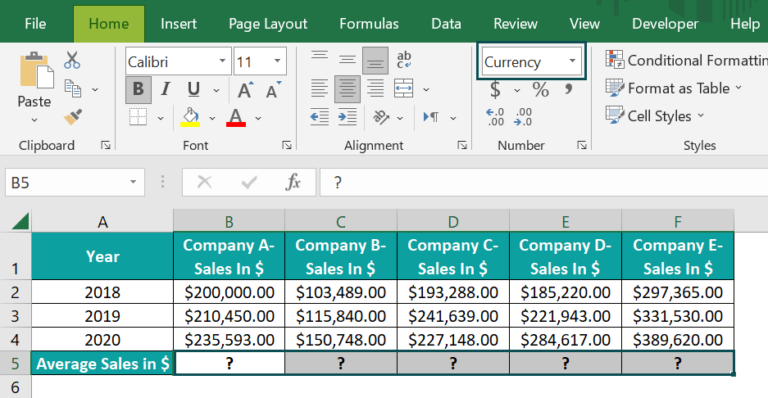Suppose the requirement is to determine the average sales of each company and display the data in row 5. Then, here is how we can use the Forward Slash (‘/’) to perform the required division operation and get the desired outcome in the target cells.

Assume the target cells’ data format set using HomeNumber Format is Currency.

• Step 1: Select the target cell B5, enter the below formula, and press Enter.
=SUM(B2:B4)/3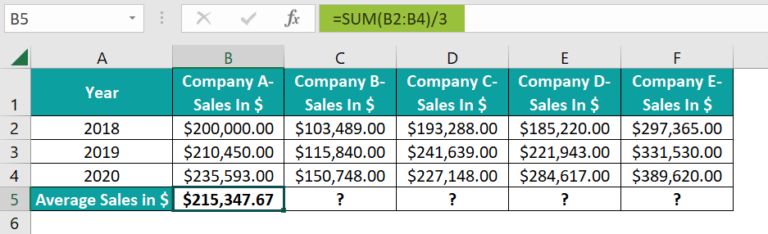• Step 2: Drag the fill handle to the right to implement the formula in cell range C5:F5.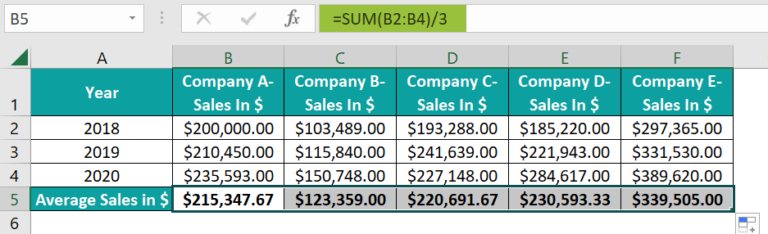Each formula in row 5 finds the sum of each company’s three years’ sales data and divides the result by 3, a constant number, to determine their average sales.